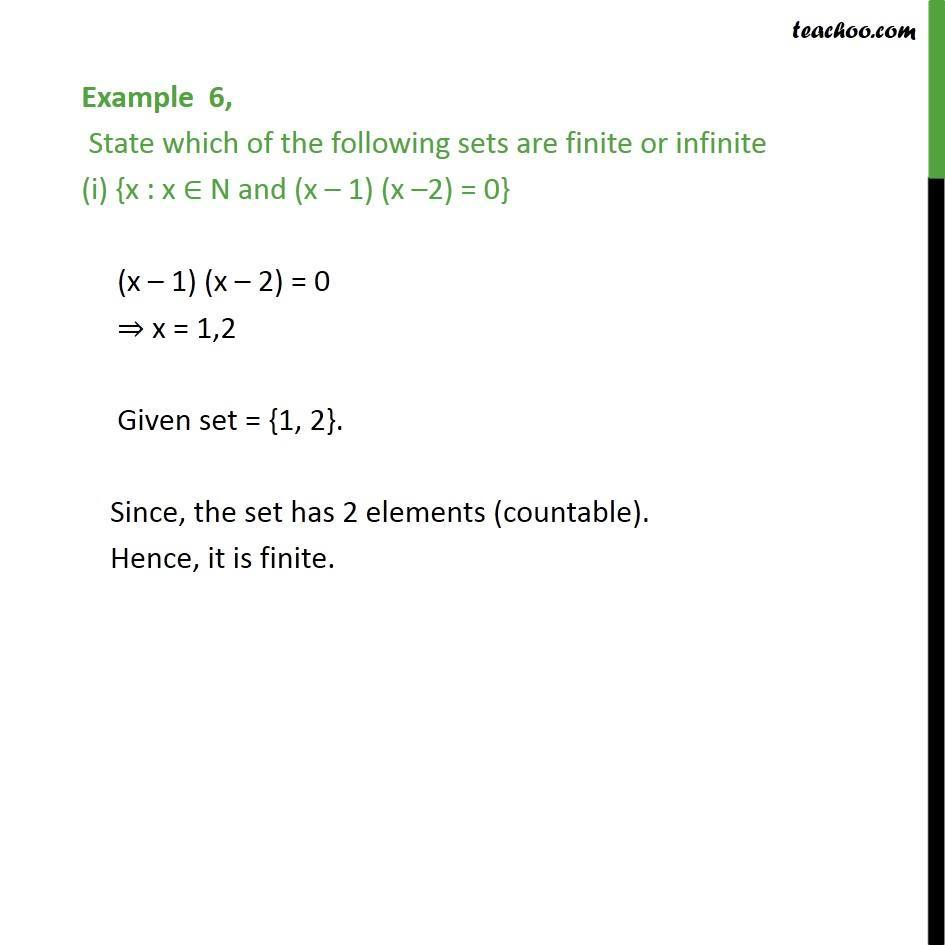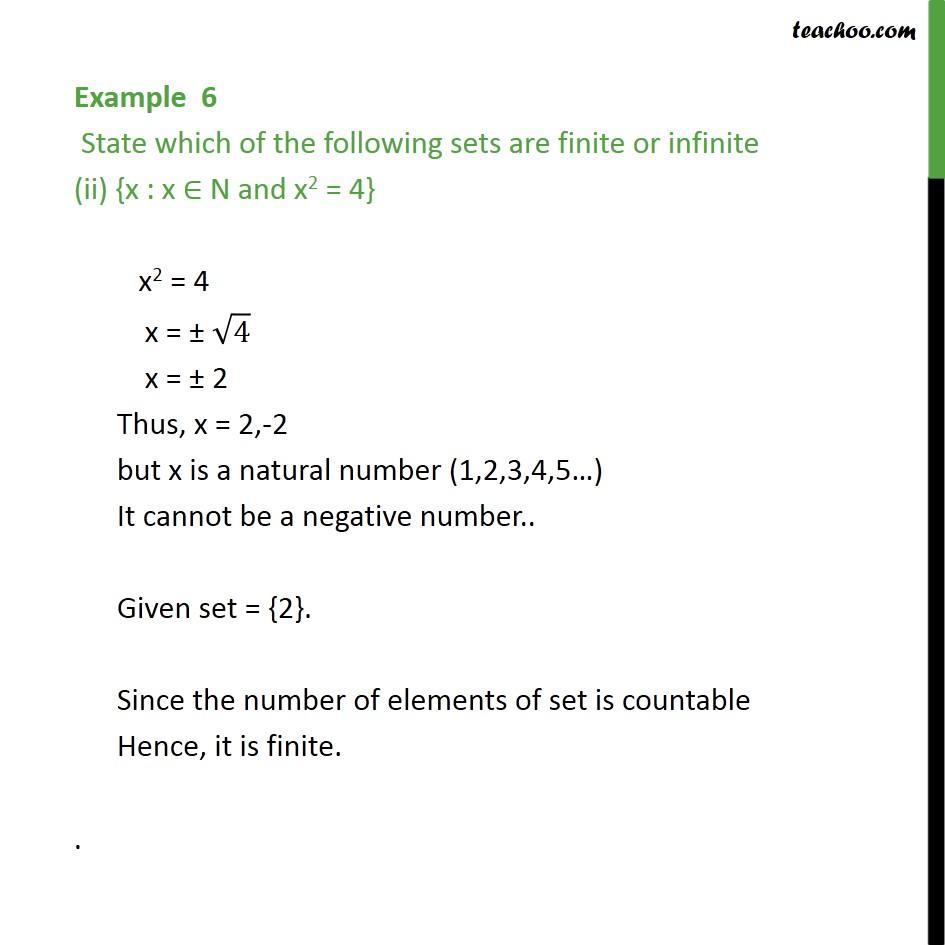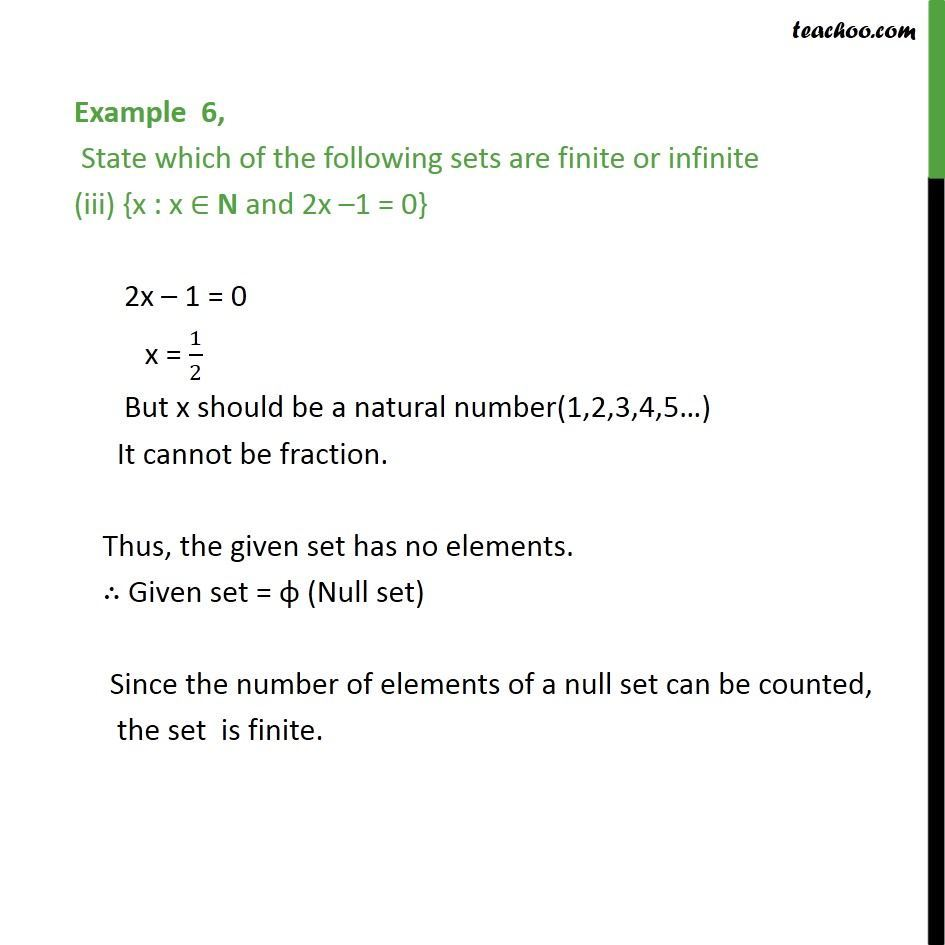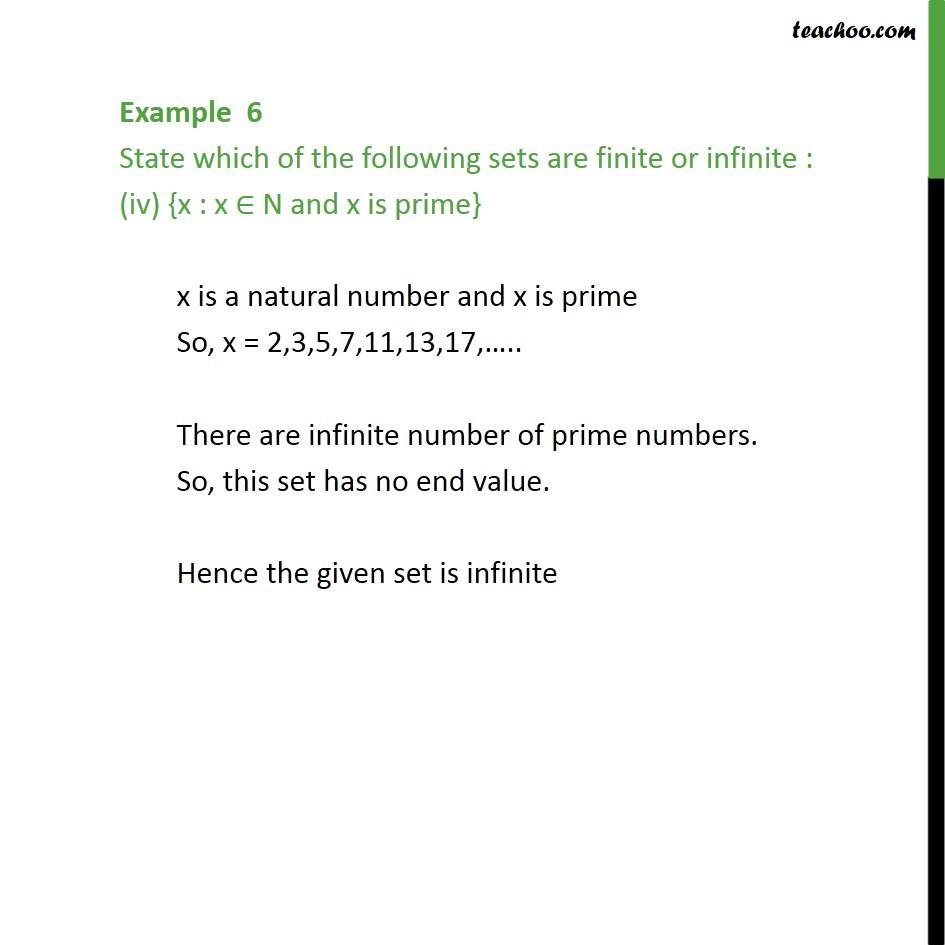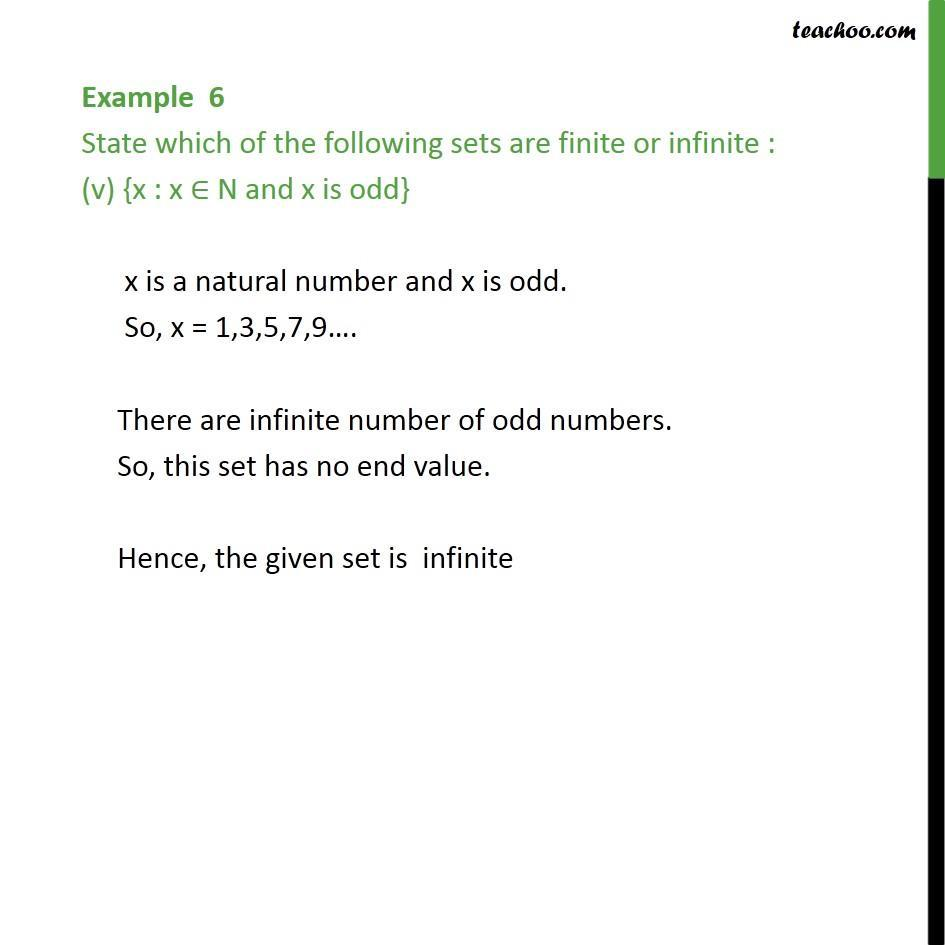1. Chapter 1 Class 11 Sets
2. Serial order wise
3. Examples

Transcript

Example 6, State which of the following sets are finite or infinite (i) {x : x ∈ N and (x – 1) (x –2) = 0} (x – 1) (x – 2) = 0 ⇒ x = 1,2 Given set = {1, 2}. Since, the set has 2 elements (countable). Hence, it is finite.

Examples

Chapter 1 Class 11 Sets
Serial order wise

About the AuthorDavneet Singh
Davneet Singh is a graduate from Indian Institute of Technology, Kanpur. He has been teaching from the past 9 years. He provides courses for Maths and Science at Teachoo.